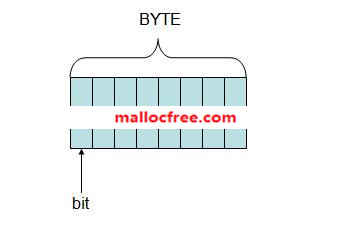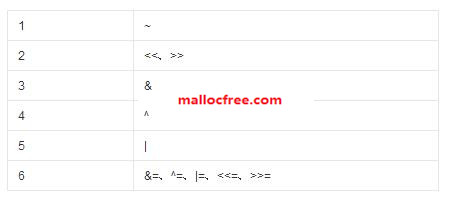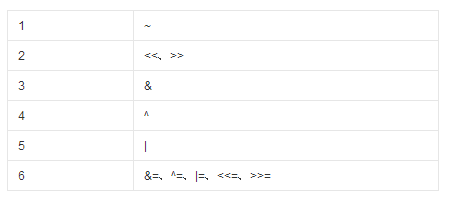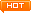﻿ 第十二章 位运算

## 12.1二进制与位运算## 12.2与（&）运算1&1 = 11&0 = 0。那么如何计算两个整数的与运算呢？比如计算15&10，首先15的二进制为：111110的二进制为1010，所以15&10为：int main(void)

{

int a = 15;

int b = 10;

int c = 15&10;

printf(“a&b=%d\n”, c);

return 0;

}

## 12.3与（&）运算的应用

int x=100;

x&7即为x的后三位。因为7的二进制码为0111。所以，任何一个数与7进行与运算，那么其它位都会被置零。只有后三位得到保留。

x&(~(1<<n))即可将xn位置为零。

#define CLEARFLAG(a,n) ((a) &= ~(1<<(n)))//把整数a中第n位置为0

x&(1<<n);

#define FLAGON(a,n) ((a)&(1<<(n)))//判断整数a中第n位是否为1

## 12.4或（|）运算1|1 = 11|0 = 1 0|0 = 0。那么如何计算两个整数的或运算呢？比如计算15|10，首先15的二进制为：111110的二进制为1010，所以15|10为：int main(void)

{

int a = 15;

int b = 10;

int c = 15|10;

printf(“a|b=%d\n”, c);

return 0;

}

## 12.5或（|）运算的应用

#define SETFLAG(a,n)  ((a) |= (1<<(n)))//把整数a中的第n位置为1

## 12.6取反（~）运算~1 = 0~0 = 1。那么如何计算1个整数的取反运算呢？比如计算~10，首先10的二进制为：1010~10为：int main(void)

{

int a = 10;

int b = ~10;

printf(“~10=%d\n”, b);

return 0;

}

## 12.7取反（~）运算的应用

#define CLEARFLAG(a,n) ((a) &= ~(1<<(n)))//把整数a中第n位置为0

## 12.8异或（^）运算1^1 = 01^0 = 1 0^0 = 0。那么如何计算两个整数的异或运算呢？比如计算15^10，首先15的二进制为：111110的二进制为1010，所以15^10为：int main(void)

{

int a = 15;

int b = 10;

int c = 15^10;

printf(“a^b=%d\n”, c);

return 0;

}

## 12.9异或（^）运算的应用

a^a==0

a^0==a

1，置零

xor eax,eax

2，交换2个数

#define SWAP(a,b) \

do{\

a=a^b;\

b=a^b;\

a=a^b;\

}while(0)

3，单指针域构造双向链表

Tail->next = PL^NULL

pl=NULL;

p=Head;

while(p!=Tail)

{

pr=pl^(p->next);

pl=p;

p=pr;

}

pr=NULL;

p=Tail;

while(p!=Head)

{

pl=pr^(p->next);

pr=p;

p=pl;

}

## 12.10左移（<<）运算

int main(void)

{

int a = 10;

int b = a<<2;

printf(“b=%d\n”, b);

return 0;

}## 12.11右移（>>）运算

12>>23

C语言中，右移运算符为算术右移运算符，即左边用符号位来填充。

int main(void)

{

int a = -3;

int b = 10;

int c = a >> 2;

int d = b >>1;

printf(“a>>2=%d, b>>1=%d\n”, c, d);

return 0;

}

## 12.12位运算优先级

位运算符的优先级如下图，位置靠前的优先级高于位置靠后的优先级。## 12.13常见位运算

1．任何一个数和0异或是它的本身：

a^0=a

a^a=0

void swap(int *a, int *b)

{

*a = *a ^ *b;

*b = *a ^ *b;

*a = *a ^ *b;

}

1）  将第n位置位或清零：

#define  BITN (1<<n)

2）  清除整数a最右边的1

问题：如何判断判断整数x的二进制中含有多少个1

分析：此题是微软公司的一道笔试题。下面用&运算来解决此题。

代码如下：

int func(int x )

{

int countx = 0;

while ( x )

{

countx ++;

x = x&(x-1);

}

return countx;

}

 功能 示例 位运算 去掉最后一位 (101101->10110) x  >>  1 在最后加一个0 (101101->1011010) x  <<  1 在最后加一个1 (101101->1011011) (x  <<  1) + 1 把最后一位变成1 (101100->101101) x  |  1 把最后一位变成0 (101101->101100) (x  |  1) - 1 最后一位取反 (101101->101100) x  ^  1 把右数第k位变成1 (101001->101101,k=3) x  |  (1 <<  (k - 1)) 把右数第k位变成0 (101101->101001,k=3) x  &  ~ (1 <<  (k - 1)) 右数第k位取反 (101001->101101,k=3) x  ^  (1  <<  (k - 1)) 取末三位 (1101101->101) x & 7 取末k位 (1101101->1101,k=5) x  &  ((1 << k) - 1) 取右数第k位 (1101101->1,k=4) x  >>  (k - 1)  & 1 把末k位变成1 (101001->101111,k=4) x  |  ((1 << k )- 1) 末k位取反 (101001->100110,k=4) x  ^  ((1 <<  k) - 1) 去掉整数最右边的1 (100101111->100101110) x  &  (x – 1) 把右边连续的1变成0 (100101111->100100000) x  &  (x + 1) 把右起第一个0变成1 (100101111->100111111) x  |  (x + 1) 把右边连续的0变成1 (11011000->11011111) x  |  (x - 1) 去掉右起第一个1的左边 (100101000->1000) x  &  (x  ^  (x - 1)) 取右边连续的1 (100101111->1111) (x  ^  (x + 1))  >>  1

## 12.14位运算在软件工程中的运用

WDK中创建文件的IRP中，有一个Options整数，它的高8位记录了对文件的修改标记。因此可以使用下面的位运算来获取这8位的值：

ulDisposition = (lpIrpStack->Parameters.Create.Options >> 24) & 0xFF;

#define SETFLAG(a,n)  ((a) |= (1<<(n)))//把整数a中的第n位置为1

#define CLEARFLAG(a,n) ((a) &= ~(1<<(n)))//把整数a中第n位置为0

#define FLAGON(a,n) ((a)&(1<<(n)))//判断整数a中第n位是否为1看文字不过瘾？点击我，进入周哥教IT视频教学